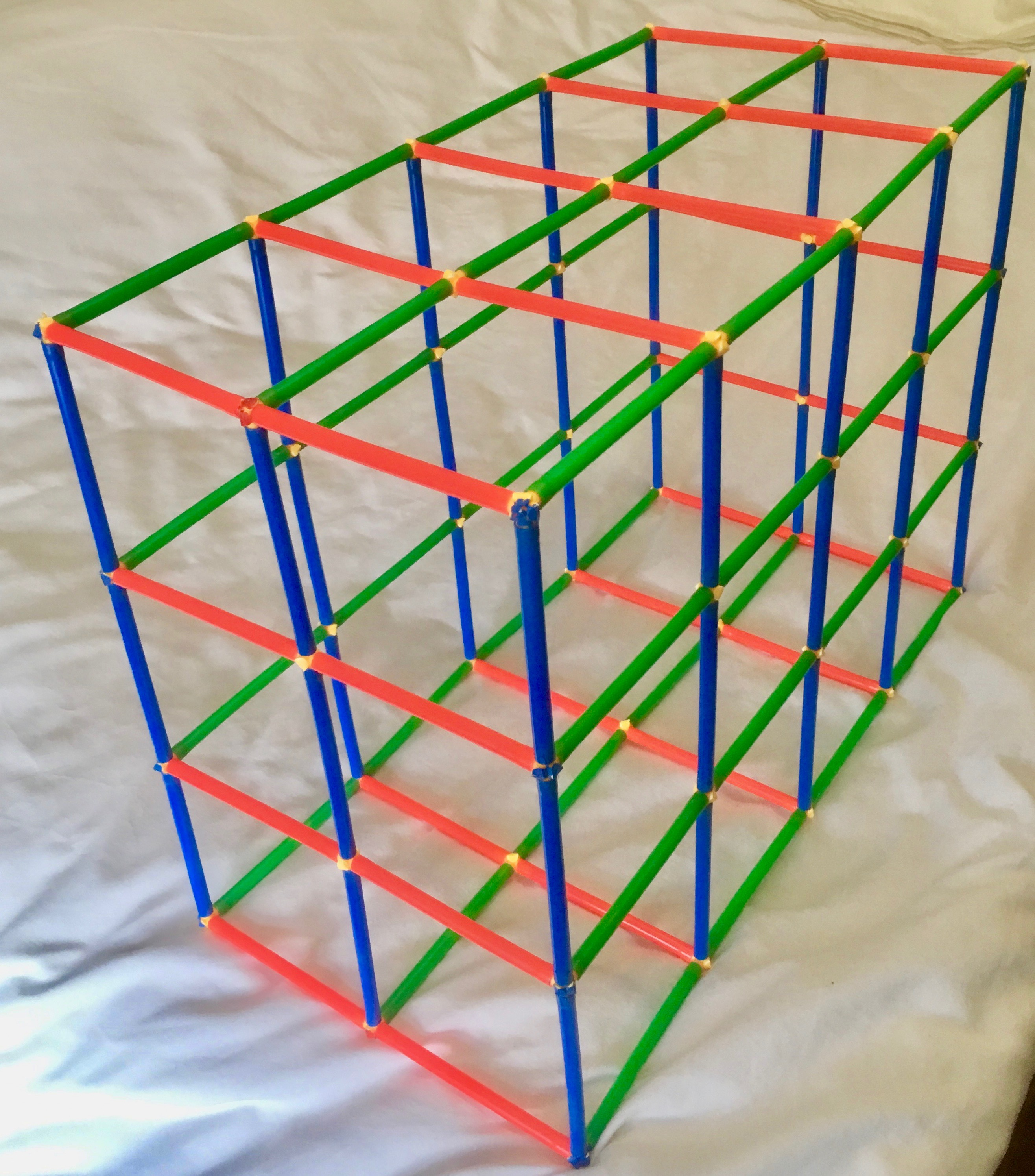# Wire Cages

#### Wire cages are used to contain large rocks in some building projects.

Here is a small version of a 2 by 3 by 4 wire cage cuboid, made with plastic straws.We can see 14 red rods of length 2 units, 12 blue rods of length 3 units and 10 green rods of length 4 units.

### Challenge 1

The example above of dimensions 2 by 3 by 4 uses exactly 36 rods and has a volume of 24 cube units.

Find all the cages that have a volume of 20 to 25 cube units inclusive and use 40 to 50 rods inclusive.

### Challenge 2

The example above of dimensions 2 by 3 by 4 uses exactly 36 rods.

Can you find a cuboid that uses exactly 28 rods?
Can you find all the cuboids that use exactly 28 rods?

### Challenge 3

Find at least one pair of cuboids of different dimensions, that when constructed:
- Use exactly 100 rods
AND
- Have each dimension greater than 2 rods.

This sheet contains a copy of the task.